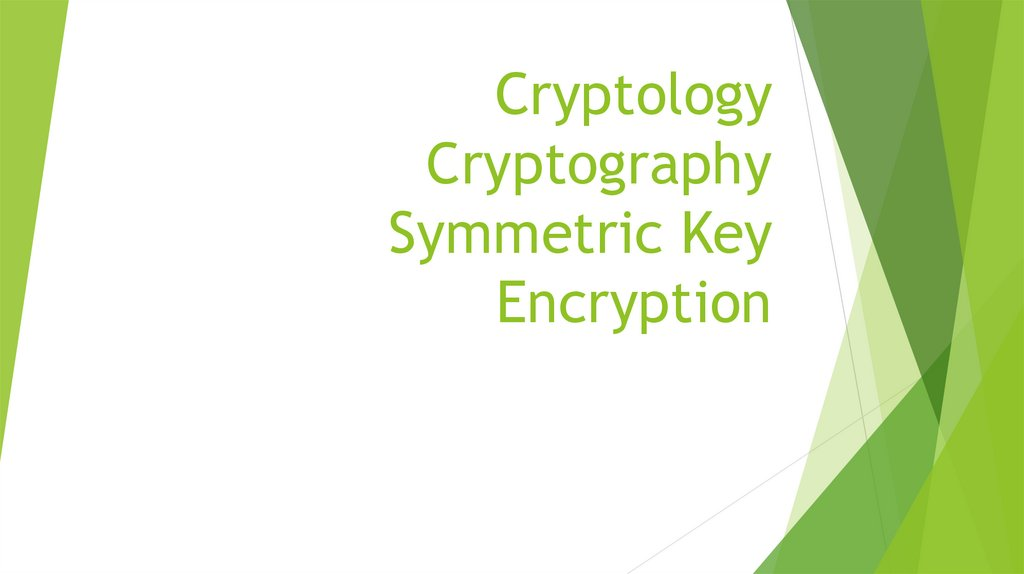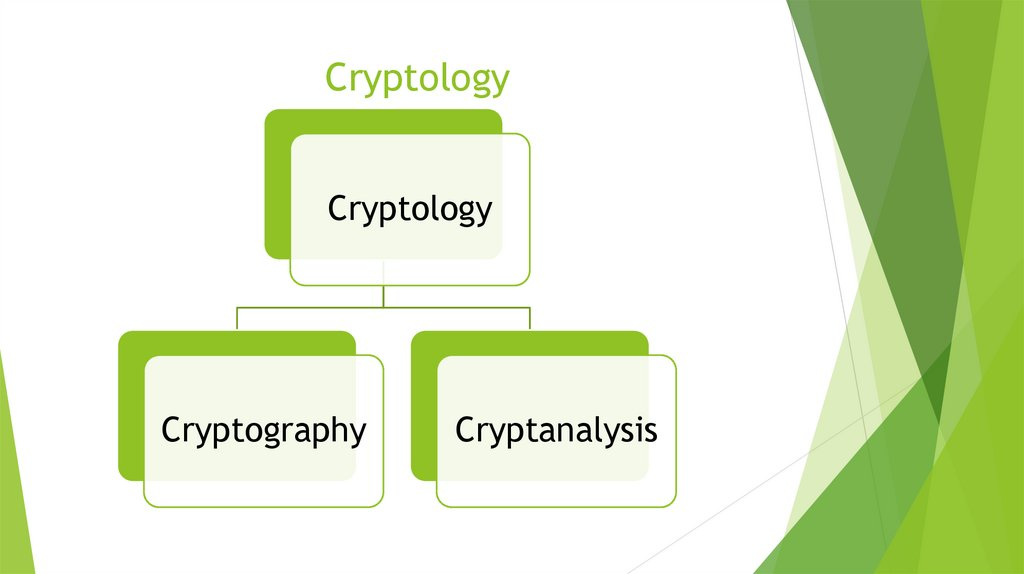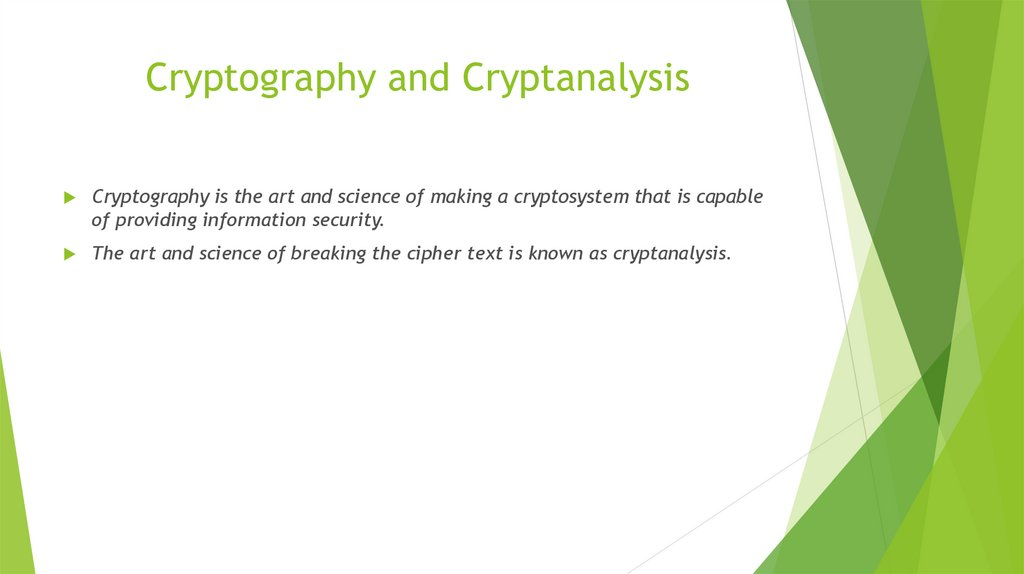# Cryptology Cryptography Symmetric Key Encryption

Cryptology
Cryptography
Cryptanalysis

## 3. Cryptography and Cryptanalysis

Cryptography is the art and science of making a cryptosystem that is capable
of providing information security.
The art and science of breaking the cipher text is known as cryptanalysis.

## 4. Cryptography Primitives

Encryption
Hash functions
Message Authentication codes (MAC)
Digital Signatures

## 5. Cryptography Primitives

Primitives
Service
Encryption
Hash Function Message
Authenticatio
n codes
Digital
Signatures
Confidentiality +
-
-
-
Data Integrity
-
+
+
+
Authentication -
-
+
+

## 7. Types of Cryptosystems

Symmetric Key Encryption
Asymmetric Key Encryption
The main difference between these cryptosystems is the relationship between
the encryption and the decryption key.

## 8. Symmetric Key Encryption

Digital Encryption Standard (DES)
Triple-DES (3DES)
IDEA
BLOWFISH

Ciphers
Substitution
Monoalphabetic
Transposition
Polyalphabetic

## 11. Modern Symmetric Key Encryption

Digital data is represented in strings of binary digits (bits)
unlike alphabets. Modern cryptosystems need to process this
binary strings to convert in to another binary string. Based
on how these binary strings are processed, a symmetric
encryption schemes can be classified in to −
Block
Ciphers
Stream
Ciphers

## 12. Block Cipher Schemes

Digital Encryption Standard (DES) − The popular block cipher of the 1990s. It is now
considered as a ‘broken’ block cipher, due primarily to its small key size.
Triple DES − It is a variant scheme based on repeated DES applications. It is still a
respected block ciphers but inefficient compared to the new faster block ciphers
available.
Advanced Encryption Standard (AES) − It is a relatively new block cipher based on the
encryption algorithm Rijndael that won the AES design competition.
IDEA − It is a sufficiently strong block cipher with a block size of 64 and a key size of 128
bits. A number of applications use IDEA encryption, including early versions of Pretty
Good Privacy (PGP) protocol. The use of IDEA scheme has a restricted adoption due to
patent issues.
Twofish − This scheme of block cipher uses block size of 128 bits and a key of variable
length. It was one of the AES finalists. It is based on the earlier block cipher Blowfish
with a block size of 64 bits.
Serpent − A block cipher with a block size of 128 bits and key lengths of 128, 192, or 256
bits, which was also an AES competition finalist. It is a slower but has more secure
design than other block cipher.

## 15. Initial permutation

The initial text T (64 bit block) is converted using the initial permutation,
which is determined by Table 1:

## 16. Encryption Cycles

Obtained after the initial permutation, the 64-bit IP (T) block participates in
16 Feistel transformation cycles.
- 16 Feistel transformation cycles:
Split IP (T) into two parts
L 0, R 0, where L 0, R 0 - respectively 32 high-order bits and 32 low-order
bits of the block
T 0 IP (T) = L 0 R 0
T i - 1 = L i - 1 R i - 1 T_ {i-1} = L_ {i-1} R_ {i-1}} the result of (i-1) iteration,
then the result of the i-th iteration T i = L i R i is determined by:
Li=Ri-1
R i = L i - 1 ⊕ f (R i - 1, k i)
The left half
L i {\ displaystyle is equal to the right half of the previous vector
L i - 1 R i - 1. And the right half R i Is
a bit-by-bit addition by modulo 2.
L i - 1 and f (R i - 1, k i)
In 16-cycles of the Feistel transformation, the function f plays the role of
encryption.

## 17. function f

Arguments of the function f are a 32-bit
vector R i – 1 and a 48-bit key k i , which is
the result of converting the 56-bit cipher
source key k .
To calculate the function f consistently used
expansion function E,
addition modulo 2 with a key k i
Transformation S, consisting of 8
transformations S –blocks S 1, S 2, S 3 ... S
8,
straight P
.

## 26. homework

cryptographic primitives: definitions, examples of use;
stream symmetric algorithms: definitions, examples,
purposes of use;